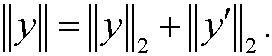3. Some function spaces.

Example 1: C[a,b] is the set of continuous functions y=y(x) on [a,b]. To be able to compare different functions we need to define a norm on the space. We can for instance useorExample 2: C1[a,b] is the set of continuous functions on [a,b] that also has continuous derivative. As norms we can here use the weak normorExample 3: The spaces Cn[a,b], n=2,3,… ar defined in a corresponding way.

<>Given a specific norm, we get the distance d(y1,y2) between two functions y1 and y2 as

<>Remark: The examples 1-3 are examples of normed linear spaces (see also part 11).